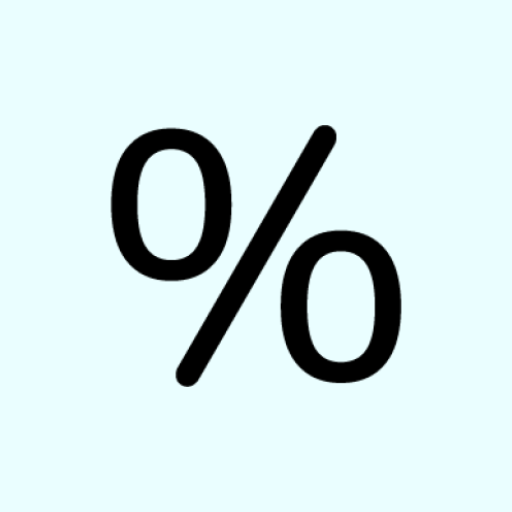# Quick Percentage Calculator on PC/Mac

Score: 4.6

OS: Windows, OS X, 4.1 +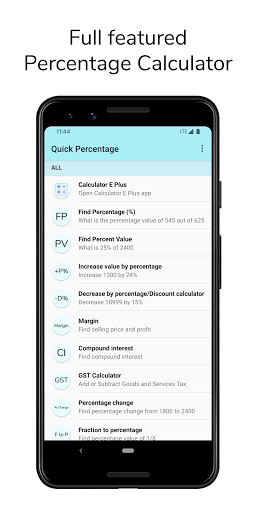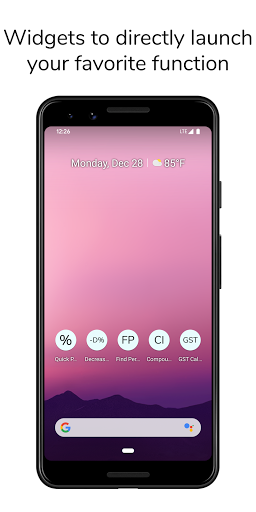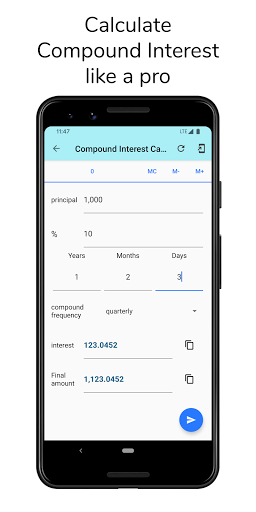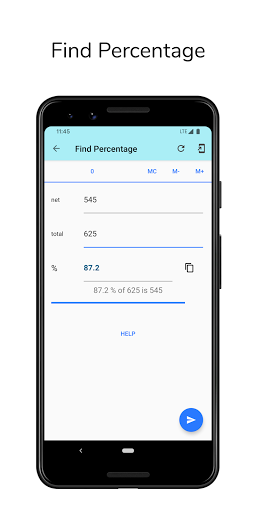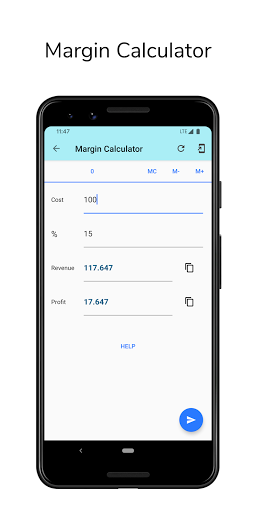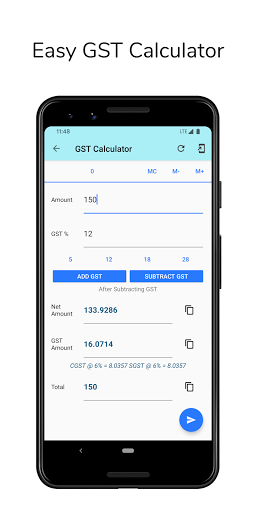Quick Percentage Calculator

Free and All in one percentage calculator app with 11 handy percentage calculators with convenient features like easy copy-paste, memory keys, dark mode, support for shortcut widgets and direct result sharing.

Quick Percentage Calculator offers

1. Compound Interest Calculator

A dedicated compound interest calculator where you can set the duration / period of interest precisely in years, months or even days.

2. Margin Calculator

Find Selling price and profit using Margin calculator.

3. GST Calculator - Calculate, Add or Remove GST amount from a product price

Calculate Goods and Service Tax of a product. Also you can add GST amount to a product price or remove GST amount from the product price.

4. Basic Calculator with History and Memory

You can directly input calculations like 23+23 , 15*30 , 40-20 , 10/5 and find results.

5. Find percentage ( % )

You can calculate simple percentage.
For example, you can calculate what is the percentage of 545 out of 625.

6. Percentage value

You can simply find percentage value of a number.
For example, you can find what is 25% of 18999.

7. Increase or decrease by percentage

Increase a number by a percentage. Ex. Increase 5400 by 25%. This function can be used to calculate taxes like GST, VAT, Excise duty, etc.

Decrease a number by a percentage. Calculate discounted price by entering original product price and dicount percentage. Ex. Decrease 25% from 1200.

8. Fraction to Percentage

Convert any fraction like 3/2 to percentage like 150%.

9. Tip and Split Calculate

You can calculate tip and per head contribution.
For example, You can divide the bill of 1000 in 8 people.

10. Price Comparison

You can compare prices of a smaller quantity product and a bigger quantity product.

11. BMI (Body Mass Index) Calculator

Calculate your Body Mass Index (BMI) using BMI Counter.
##### Recent Updates - Version 4.0.1
• ★ Improvements to User Interface